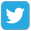Prepare forOlympiad Redeem Vouchers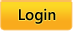Common Core:  MATH |  LANGUAGE ARTS |  SCIENCE
 Grades: Kindergarten |  First |  Second |  Third |  Fourth |  Fifth

# Common Core

Skills available for Common Core fourth-grade math standards

Click on the name of a skill to practice that skill.

### Operations and Algebraic Thinking.

Use the four operations with whole numbers to solve problems.
• CC.4.OA.1 Interpret a multiplication equation as a comparison, e.g., interpret 35 = 5 x 7 as a statement that 35 is 5 times as many as 7 and 7 times as many as 5. Represent verbal statements of multiplicative comparisons as multiplication equations.
• CC.4.OA.2 Multiply or divide to solve word problems involving multiplicative comparison, e.g., by using drawings and equations with a symbol for the unknown number to represent the problem, distinguishing multiplicative comparison from additive comparison.
• CC.4.OA.3 Solve multistep word problems posed with whole numbers and having whole-number answers using the four operations, including problems in which remainders must be interpreted. Represent these problems using equations with a letter standing for the unknown quantity. Assess the reasonableness of answers using mental computation and estimation strategies including rounding.

Gain familiarity with factors and multiples.
• CC.4.OA.4 Find all factor pairs for a whole number in the range 1-100. Recognize that a whole number is a multiple of each of its factors. Determine whether a given whole number in the range 1-100 is a multiple of a given one-digit number. Determine whether a given whole number in the range 1-100 is prime or composite.

Generate and analyze patterns.
• CC.4.OA.5 Generate a number or shape pattern that follows a given rule. Identify apparent features of the pattern that were not explicit in the rule itself. For example, given the rule “Add 3” and the starting number 1, generate terms in the resulting sequence and observe that the terms appear to alternate between odd and even numbers. Explain informally why the numbers will continue to alternate in this way.

### Number and Operations in Base Ten

Generalize place value understanding for multi-digit whole numbers.
• CC.4.NBT.1 Recognize that in a multi-digit whole number, a digit in one place represents ten times what it represents in the place to its right. For example, recognize that 700 ÷ 70 = 10 by applying concepts of place value and division. (Grade 4 expectations in this domain are limited to whole numbers less than or equal to 1,000,000.)

• CC.4.NBT.2 Read and write multi-digit whole numbers using base-ten numerals, number names, and expanded form. Compare two multi-digit numbers based on meanings of the digits in each place, using >, =, and < symbols to record the results of comparisons. (Grade 4 expectations in this domain are limited to whole numbers less than or equal to 1,000,000.)

• CC.4.NBT.3 Use place value understanding to round multi-digit whole numbers to any place. (Grade 4 expectations in this domain are limited to whole numbers less than or equal to 1,000,000.)

Use place value understanding and properties of operations to perform multi-digit arithmetic.
• CC.4.NBT.4 Fluently add and subtract multi-digit whole numbers using the standard algorithm. (Grade 4 expectations in this domain are limited to whole numbers less than or equal to 1,000,000. A range of algorithms may be used.)

• CC.4.NBT.5 Multiply a whole number of up to four digits by a one-digit whole number, and multiply two two-digit numbers, using strategies based on place value and the properties of operations. Illustrate and explain the calculation by using equations, rectangular arrays, and/or area models. (Grade 4 expectations in this domain are limited to whole numbers less than or equal to 1,000,000. A range of algorithms may be used.)
• CC.4.NBT.6 Find whole-number quotients and remainders with up to four-digit dividends and one-digit divisors, using strategies based on place value, the properties of operations, and/or the relationship between multiplication and division. Illustrate and explain the calculation by using equations, rectangular arrays, and/or area models. (Grade 4 expectations in this domain are limited to whole numbers less than or equal to 1,000,000. A range of algorithms may be used.)

### Number and Operations—Fractions

Extend understanding of fraction equivalence and ordering.
• CC.4.NF.1 Explain why a fraction a/b is equivalent to a fraction (n × a)/(n × b) by using visual fraction models, with attention to how the number and size of the parts differ even though the two fractions themselves are the same size. Use this principle to recognize and generate equivalent fractions. (Grade 4 expectations in this domain are limited to fractions with denominators 2, 3, 4, 5, 6, 8, 10, 12, and 100.)
• CC.4.NF.2 Compare two fractions with different numerators and different denominators, e.g., by creating common denominators or numerators, or by comparing to a benchmark fraction such as 1/2. Recognize that comparisons are valid only when the two fractions refer to the same whole. Record the results of comparisons with symbols >, =, or <, and justify the conclusions, e.g., by using a visual fraction model. (Grade 4 expectations in this domain are limited to fractions with denominators 2, 3, 4, 5, 6, 8, 10, 12, and 100.)

Build fractions from unit fractions by applying and extending previous understandings of operations on whole numbers.
• CC.4.NF.3 Understand a fraction a/b with a > 1 as a sum of fractions 1/b. (Grade 4 expectations in this domain are limited to fractions with denominators 2, 3, 4, 5, 6, 8, 10, 12, and 100.)
• CC.4.NF.3a Understand addition and subtraction of fractions as joining and separating parts referring to the same whole.

• CC.4.NF.3b Decompose a fraction into a sum of fractions with the same denominator in more than one way, recording each decomposition by an equation. Justify decompositions, e.g., by using a visual fraction model. Examples: 3/8 = 1/8 + 1/8 + 1/8 ; 3/8 = 1/8 + 2/8 ; 2 1/8 = 1 + 1 + 1/8 = 8/8 + 8/8 + 1/8.
• CC.4.NF.3c Add and subtract mixed numbers with like denominators, e.g., by replacing each mixed number with an equivalent fraction, and/or by using properties of operations and the relationship between addition and subtraction.

• CC.4.NF.3d Solve word problems involving addition and subtraction of fractions referring to the same whole and having like denominators, e.g., by using visual fraction models and equations to represent the problem.

Build fractions from unit fractions by applying and extending previous understandings of operations on whole numbers.
• CC.4.NF.4 Apply and extend previous understandings of multiplication to multiply a fraction by a whole number. (Grade 4 expectations in this domain are limited to fractions with denominators 2, 3, 4, 5, 6, 8, 10, 12, and 100.)
• CC.4.NF.4a Understand a fraction a/b as a multiple of 1/b. For example, use a visual fraction model to represent 5/4 as the product 5 × (1/4), recording the conclusion by the equation 5/4 = 5 × (1/4).
• CC.4.NF.4b Understand a multiple of a/b as a multiple of 1/b, and use this understanding to multiply a fraction by a whole number. For example, use a visual fraction model to express 3 × (2/5) as 6 × (1/5), recognizing this product as 6/5. (In general, n × (a/b) = (n × a)/b.)
• CC.4.NF.4c Solve word problems involving multiplication of a fraction by a whole number, e.g., by using visual fraction models and equations to represent the problem. For example, if each person at a party will eat 3/8 of a pound of roast beef, and there will be 5 people at the party, how many pounds of roast beef will be needed? Between what two whole numbers does your answer lie?

Understand decimal notation for fractions, and compare decimal fractions.
• CC.4.NF.5 Express a fraction with denominator 10 as an equivalent fraction with denominator 100, and use this technique to add two fractions with respective denominators 10 and 100. For example, express 3/10 as 30/100 and add 3/10 + 4/100 = 34/100. (Students who can generate equivalent fractions can develop strategies for adding fractions with unlike denominators in general. But addition and subtraction with unlike denominators in general is not a requirement at this grade.) (Grade 4 expectations in this domain are limited to fractions with denominators 2, 3, 4, 5, 6, 8, 10, 12, and 100.)

• CC.4.NF.6 Use decimal notation for fractions with denominators 10 or 100. For example, rewrite 0.62 as 62/100 ; describe a length as 0.62 meters; locate 0.62 on a number line diagram. (Grade 4 expectations in this domain are limited to fractions with denominators 2, 3, 4, 5, 6, 8, 10, 12, and 100.)

• CC.4.NF.7 Compare two decimals to hundredths by reasoning about their size. Recognize that comparisons comparisons are valid only when two decimals refer to the same whole. Record the results of comparisons with the symbols >, =, or <, and justify the conclusions, e.g., by using a visual model. (Grade 4 expectations in this domain are limited to fractions with denominators 2, 3, 4, 5, 6, 8, 10, 12, and 100.)

### Measurement and Data

Solve problems involving measurement and conversion of measurements from a larger unit to a smaller unit.
• CC.4.MD.1 Know relative sizes of measurement units within one system of units including km, m, cm; kg, g; lb, oz.; l, ml; hr, min, sec. Within a single system of measurement, express measurements in a larger unit in terms of a smaller unit. Record measurement equivalents in a two-column table. For example: Know that 1 ft is 12 times as long as 1 in. Express the length of a 4 ft snake as 48 in. Generate a conversion table for feet and inches listing the number pairs (1, 12), (2, 24), (3, 36), ….

• CC.4.MD.2 Use the four operations to solve word problems involving distances, intervals of time, liquid volumes, masses of objects, and money, including problems involving simple fractions or decimals, and problems that require expressing measurements given in a larger unit in terms of a smaller unit. Represent measurement quantities using diagrams such as number line diagrams that feature a measurement scale.

• CC.4.MD.3 Apply the area and perimeter formulas for rectangles in real world and mathematical problems. For example, find the width of a rectangular room given the area of the flooring and the length, by viewing the area formula as a multiplication equation with an unknown factor.

Represent and interpret data.
• CC.4.MD.4 Make a line plot to display a data set of measurements in fractions of a unit (1/2, 1/4, 1/8). Solve problems involving addition and subtraction of fractions by using information presented in line plots. For example, from a line plot find and interpret the difference in length between the longest and shortest specimens in an insect collection.

Geometric measurement: understand concepts of angle and measure angles.
• CC.4.MD.5 Recognize angles as geometric shapes that are formed wherever two rays share a common endpoint, and understand concepts of angle measurement: -- a. An angle is measured with reference to a circle with its center at the common endpoint of the rays, by considering the fraction of the circular arc between the points where the two rays intersect the circle. An angle that turns through 1/360 of a circle is called a “one-degree angle,” and can be used to measure angles. -- b. An angle that turns through n one-degree angles is said to have an angle measure of n degrees.
• CC.4.MD.6 Measure angles in whole-number degrees using a protractor. Sketch angles of specified measure.
• CC.4.MD.7 Recognize angle measure as additive. When an angle is decomposed into non-overlapping parts, the angle measure of the whole is the sum of the angle measures of the parts. Solve addition and subtraction problems to find unknown angles on a diagram in real world and mathematical problems, e.g., by using an equation with a symbol for the unknown angle measure.

### Geometry

Draw and identify lines and angles, and classify shapes by properties of their lines and angles.
• CC.4.G.1 Draw points, lines, line segments, rays, angles (right, acute, obtuse), and perpendicular and parallel lines. Identify these in two-dimensional figures.

• CC.4.G.2 Classify two-dimensional figures based on the presence or absence of parallel or perpendicular lines, or the presence or absence of angles of a specified size. Recognize right triangles as a category, and identify right triangles.
• CC.4.G.3 Recognize a line of symmetry for a two-dimensional figure as a line across the figure such that the figure can be folded along the line into matching parts. Identify line-symmetric figures and draw lines of symmetry.

 Connect and Follow About Intelliseeds Learning My Account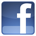Facebook Benefits Membership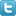Twitter Plans and Pricing Skills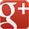Google+ FAQ Wall of Fame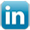LinkedIn Newsletter Student Reports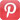Pinterest Careers Rewards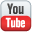Youtube Contact Us What is IntelliAbility?Our Partners Terms & Conditions Tell a FriendNon Profit Partners Testimonials User Guide PTA Fundraising Offers Common Core Math Schools Common Core ELA Virginia SOL Content Team
 Welcome to Intelliseeds Learning Smart learning for your child Intelliseeds learning provides practice in several subjects including Math, Reading Comprehension, Language Arts, Logical Reasoning, Mental Ability, Analytical Ability and Critical Thinking, which are essential to the success of every student. Skills are organized by grades, topics, practice tests and time bound tests. The Math section covers skills such as counting, number operations, algebra, story problems and many more. The IntelliAbility section focuses on thinking skills, patterns, sequences, problem analysis, analogies and over 50 other topics to help develop your child's brain. Click here to learn more about IntelliAbility. The Language Arts section includes reading comprehension, nouns, verbs, adverbs, adjectives, predicates, vowel sounds and many more grammar skills.Privacy | © 2022 Intelliseeds Learning™